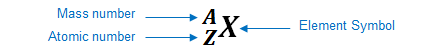# Problem: Identify the following elements. 80202 X

###### FREE Expert Solution

We’re being asked to determine the identity of the given element with the following notation:

${}_{\mathbf{80}}{}^{\mathbf{202}}\mathbf{X}$

Recall: an element can be represented as:You can determine the identity of the element using its atomic number:

Atomic number (Z):

94% (113 ratings)###### Problem Details

Identify the following elements.

80202 X